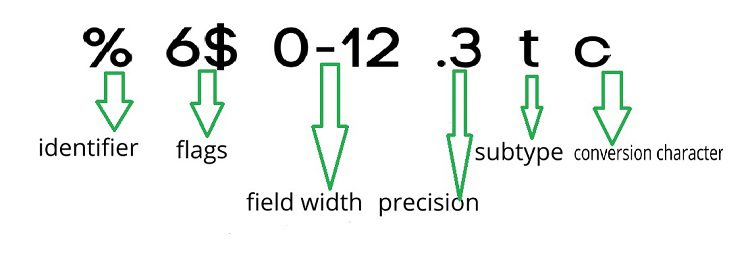# Text Formatting in MATLAB

• Difficulty Level : Expert
• Last Updated : 19 Mar, 2022

In the real-world data can be any form. This data may be in binary, numeric, text, array, and so on. And this data can be converted into text. In Matlab, the text can be formatted using a formatting operator along with formatting functions such as sprintf, numstr, fprintf, compose. These functions/operators control the text notation, significant digit, text alignment, and so on.In this article, we will see, how you can format the text in MATLAB.

A formatting operator has six criteria that include a numeric identifier, flags, field width, precision, subtype, and a conversion character.  In the figure there is space between the operator but while writing code, make sure there are no space characters allowed in the operator. Out of the six field conversion character is only the required field which means it cannot be ignored while formatting text in Matlab. And this conversion character must start by a % percent sign.

### Fields of Formatting Operator:

Conversion Character: Just like C programming, Matlab requires a format specifier so that it specifies the notation of the output. The format specifier and meaning is given below:

Example 1:

## Matlab

 `% MATLAB program for sprintf()` `A = 57*ones(1,5);` `txt = sprintf(``'%d %f %o %X %u'``, A)`

Output:

`txt = 57 57.00000 71 39 57`

Subtype: Before the conversion character, the subtype is the field is represented by a single alphabetic character. It mainly has two types:

Example 2:

## Matlab

 `% MATLAB code for subtype` `subtype_ = 19;  ` `final_sub = sprintf(``'%tu'``,subtype_)`

Output:

`final_sub = 1100480512`

Precision: The next field of formatting operators is a precision field. It is usually denoted with dot notation, it is a nonnegative integer that immediately follows a period.

Example 3:

## Matlab

 `% MATLAB code for check Precision` `check_decimal = sprintf(``'%g   %.2g   %f   %.2f'``, [1 2 3 4])`

Output:

`check_decimal = 1 2 3.000000 4.00`

We took two g and 2 f format specifiers and can see what a Precision does to the decimal point.

Field Width: A Field Width is a non-negative integer that specifies the number of digits or characters in the output. Specify different field widths. To show the width for each output, use the | character. For example, in the operator %5.1f, the field width is 5 and 0.1 precision.

Example 4:

## Matlab

 `% MATLAB code for check Field Width` `text = sprintf(``'|%e|%6e|%f|%12f|'``,[0.455312 12345 2223 0.111123])`

Output:

`text = '|4.553120e-01|1.2345e+04|2223000000|      0.111123|'`

Flags: The flags field is optional. If used then it mainly controls the formatting of the output. Additionally, it is used to describe padding, text alignment, and spacing.

Identifier: Identifiers are declared after the % and before the \$ special characters. It is an integer value to produce the output in a specified order. sprintf by default prints in sequential order, using Identifier we can arrange output in the custom order.

Example 5:

## Matlab

 `% MATLAB code for identifier` `sprintf(``'%3\$s %2\$s %1\$s'``,...` `        ``'Geeks'``,``'Premier'``,``'League'``)`

Output:

`ans = 'League Premier Geeks'`

### Formatting Text With compose():

The compose function is used to format the array data into multiple strings.

Syntax:

```var_name = compose(format_specifier,var_name)
str = compose(format_specifier,A,b,...,N)```

Example:

## Matlab

 `% MATLAB code for compose()` `compose_str1 = compose(``"%.5f"``,45.566)` `compose_str2 = compose(``"%.1f"``,45.566)`

Output:

```"compose_str1 = 45.56600"
"compose_str1 = 45.6"```

### Formatting Text With num2str():

The num2str function is used to format the number into a character array.

Syntax:

```new_var = num2str(int_var)
new_var = num2str(int_var,precision)
new_var = num2str(int_var,format_specifier)```

Example:

## Matlab

 `% MATLAB code for num2str()` `num = 92;` `chr = num2str(num)`

Output:

`chr = '92'`

My Personal Notes arrow_drop_up
Recommended Articles
Page :